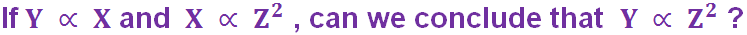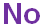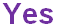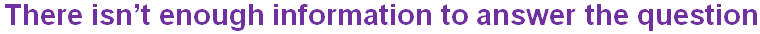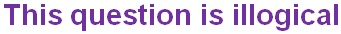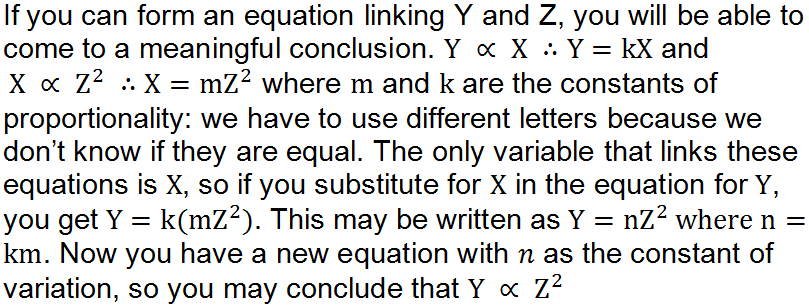Cruise down to question 4 for a question about travelling!

# Direct and Inverse Variation (H)

What happens to the area of a square if you double the length of each side? Or the volume of a balloon, if the radius doubles? Questions like this can be modelled using variation – how does one variable change in relation to another one? There is a standard approach to solving these types of problems, see how good you are in this GCSE Maths quiz on direct and inverse variation.

We can recognise questions on variation by some of the words and phrases used – ‘directly proportional’ indicates that one variable increases when the other one also increases. If you see ‘inversely proportional’ then this means one variable decreases as the other one increases. As soon as you see this in a question you can write down a statement using the proportionality symbol, ∝.

The next stage is to introduce the constant of proportionality, usually the letter k, and turn this into an equation. At this point if you have been given numerical data, you can substitute it into the equation, to find a value for k.

It is also a good idea to know what shape graph each type of variation would have. In direct proportion problems, the curve or line always goes through the origin. Inverse proportion problems have a reciprocal graph, to indicate the decrease of one variable.

Question 1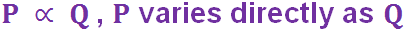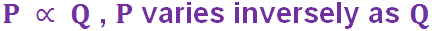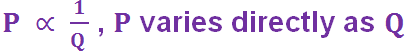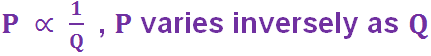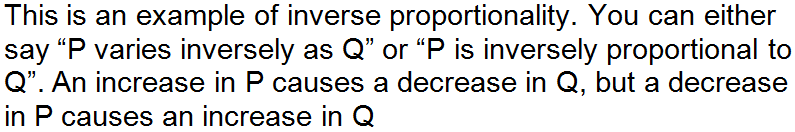Question 2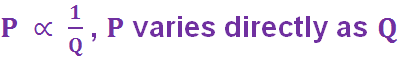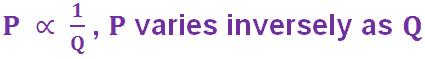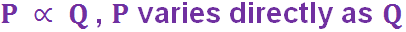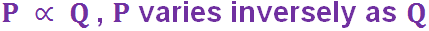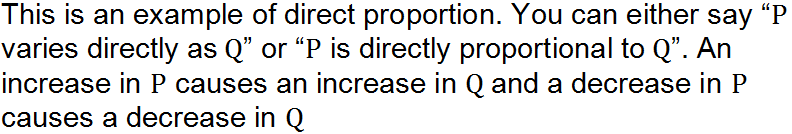Question 3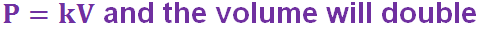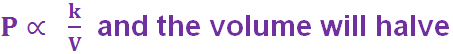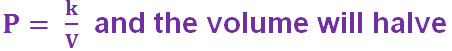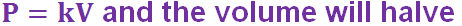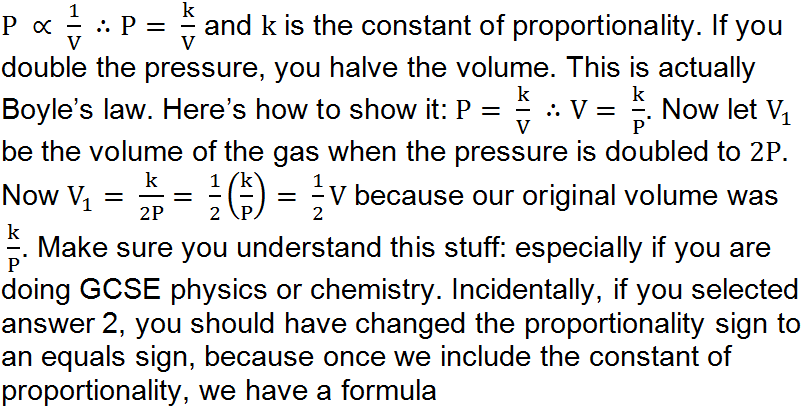Question 4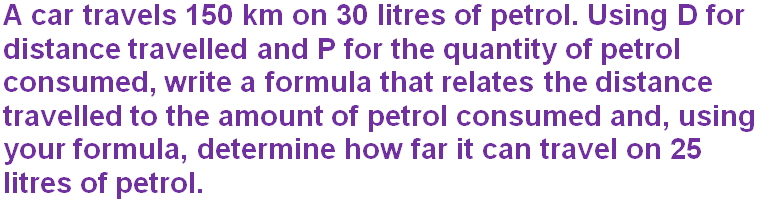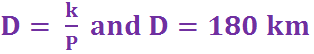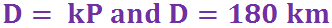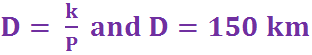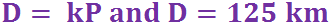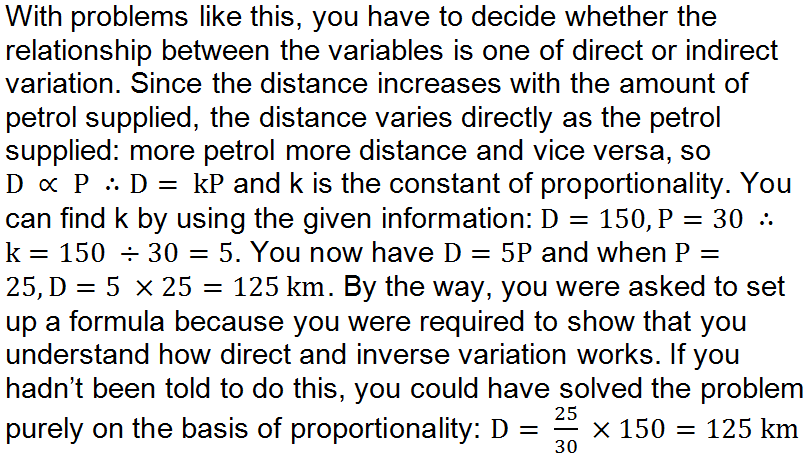Question 5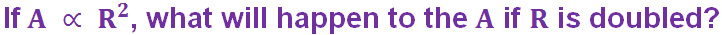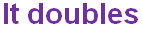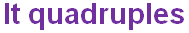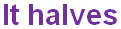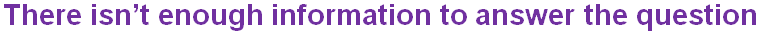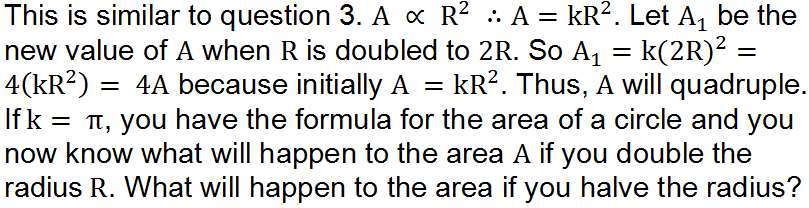Question 6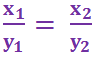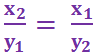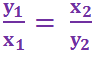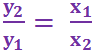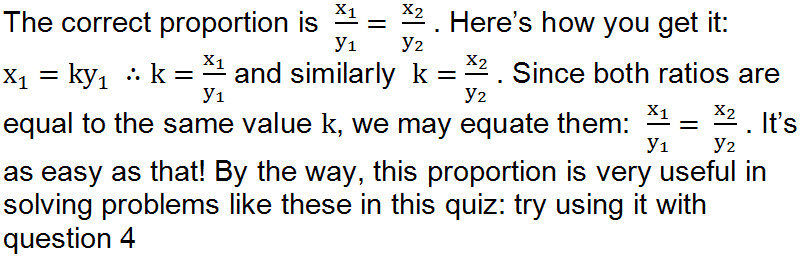Question 7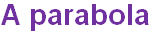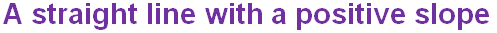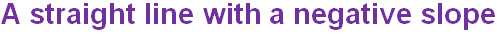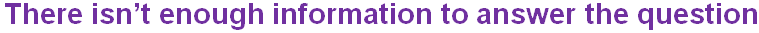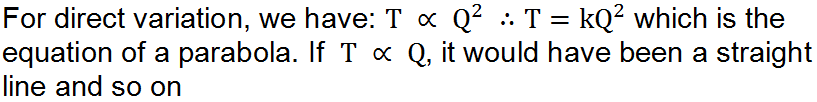Question 8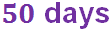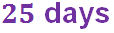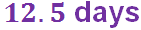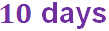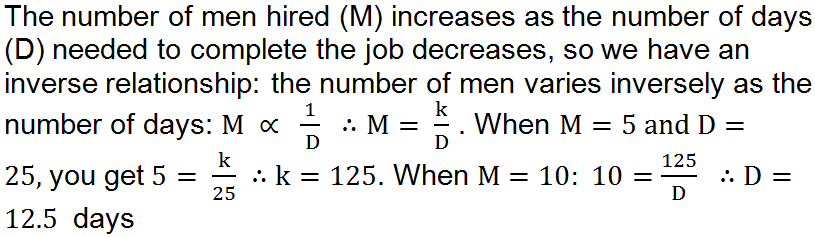Question 9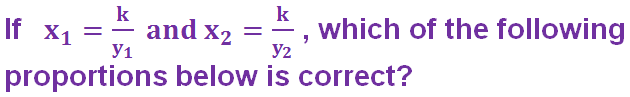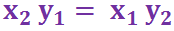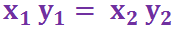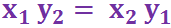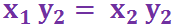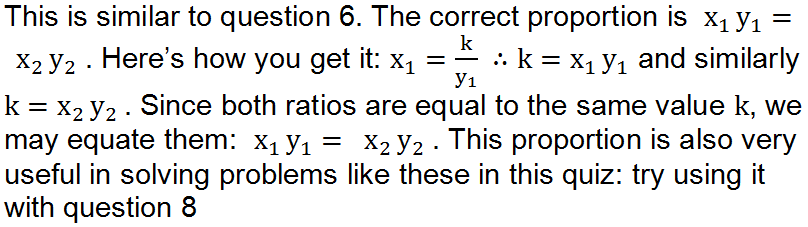Question 10